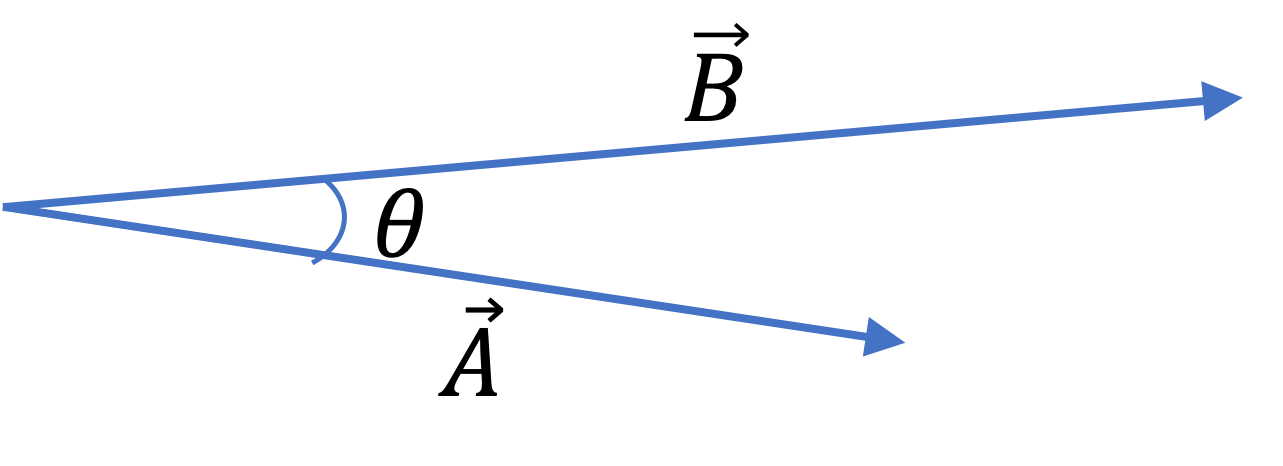## Section3.6Scalar Product Between Vectors

Scalar or dot product of two vectors results in a single real number. To be specific, consider two vectors $\vec A$ and $\vec B$ that have an angle $\theta$ between them as shown in Figure 3.6.1. We denote the scalar product by placing a dot between the abstract symbols $\vec A$ and $\vec B$ for the two vectors, as $\vec A\cdot\vec B\text{.}$Figure 3.6.1. Vectors for definition of dot product of vectors. The angle between vectors are denoted by drawing the two vectors with their tails at the same point.

The result of scalar product between $\vec A$ and $\vec B$ can be given in two equivalent ways: in terms of components of the vectors or in terms of magnitudes and angle.

\begin{align} \amp \text{(1) }\vec A \cdot\vec B = A_x B_x + A_y B_y + A_z B_z,\label{eq-dot-product-from-components}\tag{3.6.1}\\ \amp \text{(2) } \vec A \cdot\vec B = A B \cos\theta,\label{eq-dot-product-from-mag-and-angle}\tag{3.6.2} \end{align}

where $A$ and $B$ are magnitudes of these vectors.

\begin{align*} \amp A = \sqrt{A_x^2 + A_y^2 + A_z^2}\\ \amp B = \sqrt{B_x^2 + B_y^2 + B_z^2} \end{align*}

An important use of these formulas to find the angle between two vectors, given their components. Equating these Eqs. (3.6.1) and (3.6.2), and solving for $\cos\theta$ we get

\begin{equation} \cos\theta = \dfrac{A_x B_x + A_y B_y + A_z B_z}{A\, B}.\tag{3.6.3} \end{equation}

Since components are simple numbers, their multiplication order does not matter, i.e., $A_xB_x = B_xA_x\text{,}$ therefore, the order of vectors in the dot product does not matter. That is,

\begin{equation} \vec A \cdot \vec B = \vec B \cdot \vec A.\tag{3.6.4} \end{equation}

This will not be the case with the other product between vectors, called cross product, we will define below - we will find that reversing the order introduces a negative sign.

Consider the following vectors given in their component forms in a three-dimensional space, $\vec F = (3 \text{ N}, 4 \text{ N}, -5 \text{ N})\text{,}$ and $\vec d = (-4 \text{ m}, 5 \text{ m}, 3 \text{ m})\text{.}$ (a) Find the magnitudes of the two vectors. (b) Find the dot product of the two vectors. (c) What is the angle betwen the two vectors?

Hint

(a) Use $F = \sqrt{F_x^2 + F_y^2 + F_z^2}\text{,}$ (b) Use dot product based on components, (c) Use dot product based on magnitude.

(a) $7.07\text{ N} \text{,}$ $7.07\text{ m} \text{,}$ (b) $- 7\text{ N.m} \text{,}$ (c) $98^{\circ}$

Solution 1 (a)

(a) Using $F = \sqrt{F_x^2 + F_y^2 + F_z^2}$ on $\vec F$ and similar formula on $\vec d$ we immediately get the answer. The only thing we need to notice that we will also get units.

\begin{align*} F \amp = \sqrt{F_x^2 + F_y^2 + F_z^2} \\ \amp = \sqrt{(3 \text{ N})^2 + (4 \text{ N})^2 + (-5 \text{ N})^2} = 7.07\text{ N}. \end{align*}

Similarly, $d = 7.07\text{ m}\text{.}$

Solution 2 (b)

(b) Since, vectors to be multiplied are given in the component forms, we use the definition of the scalar product based on the components.

\begin{align*} \vec F \cdot \vec d \amp = F_x d_x + F_y d_y + F_z d_z\\ \amp = (3 \text{ N})\times(-4 \text{ m}) + (4 \text{ N})\times(5 \text{ m}) + (-5 \text{ N})\times(3 \text{ m}) = - 7 \text{ N.m}. \end{align*}
Solution 3 (c)

(c) Since two definitions of scalar product would give the same value and one of them has the angle $\theta$ we seek. Therefore, we solve for $\theta$ to get

\begin{equation*} \cos\theta = \dfrac{\vec F \cdot \vec d}{ F \, d}. \end{equation*}

Now, using the numerical values, we find that

\begin{equation*} \cos\theta = \dfrac{- 7 \text{ N.m}}{ 7.07\text{ N} \times 7.07\text{ m}} = -0.14. \end{equation*}

Inverting this we get

\begin{equation*} \theta = 1.71 \text{ rad or } 98^{\circ}. \end{equation*}

When will the dot product between two non-zero vectors be zero?

Hint

Dot product is zero when vectors are perpendicular to each other, i.e., $\theta = 90^{\circ}\text{.}$

Solution

Let $A$ and $B$ be the magnitudes of the vectors and $\theta$ be the angle between them. Then, we want

\begin{equation*} A\, B\, \cos\,\theta = 0 \text{ when } A\ne 0 \text{ and } B\ne 0. \end{equation*}

That means,

\begin{equation*} \cos\, \theta = 0. \end{equation*}

Since, $\theta$ can be at most $180^{\circ}\text{,}$ there is only one value at which $\cos\, \theta = 0\text{.}$ That is, when

\begin{equation*} \theta = 90^{\circ}. \end{equation*}

That is, the dot product is zero when the two vector are perpendicular.

Find the dot products:

(a) between $\vec A =$ ( $30$ m/s, due East) and $\vec B =$ ( $40$ m/s, due $60^{\circ}$ North of East),

(b) between $\vec F =$ ( $100$ N, due East) and $\vec d =$ ( $40$ m, due $120^{\circ}$ Counterclockwise from East, i.e., towards North and $30^{\circ}$ past North).

Hint

Use $A B \cos\, \theta$

(a) $600\text{ m}^2/\text{s}^2\text{,}$ (b) $- 2000\text{ N.m}\text{.}$